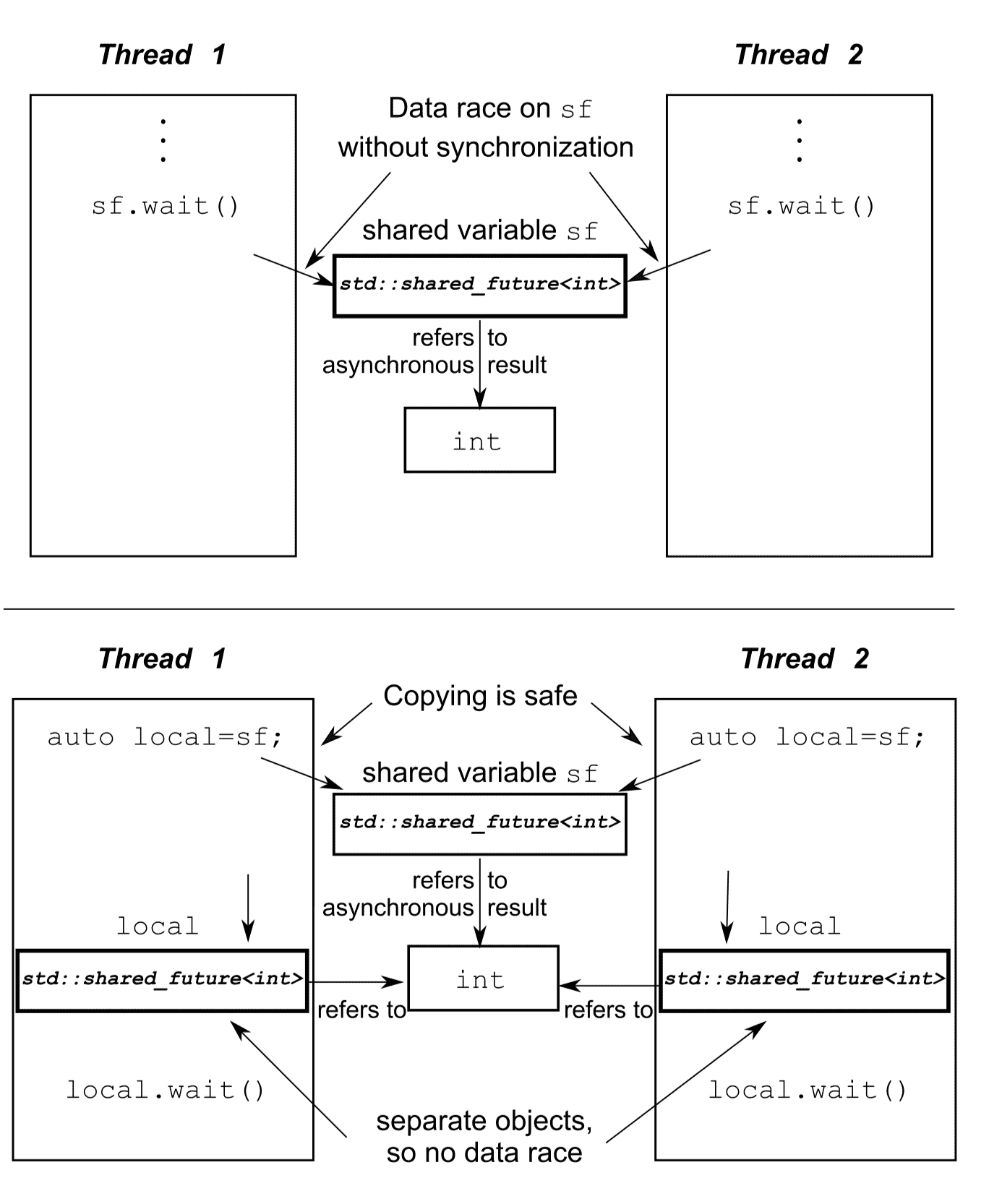# 等待事件或条件

## 等待条件达成

C++ 标准库对条件变量有两套实现：std::condition_variable 和 std::condition_variable_any，这两个实现都包含在 condition_variable.h 头文件的声明中。两者都需要与互斥量一起才能工作，前者仅能与 std::mutex 一起工作，而后者可以和合适的互斥量一起工作，从而加上了 _any 的后缀。因为std::condition_variable_any 更加通用，不过在性能和系统资源的使用方面会有更多的开销，所以通常会将 std::condition_variable 作为首选类型。当对灵活性有要求时，才会考虑 std::condition_variable_any。

std::mutex mut;
std::queue<data_chunk> data_queue;
std::condition_variable data_cond;

while(more_data_to_prepare()) {
data_chunk const data = prepare_data();
std::lock_guard<std::mutex> lk(mut);
data_queue.push(data);
data_cond.notify_one();
}
}

while (true) {
std::unique_lock<std::mutex> lk(mut);
data_cond.wait(lk, []{return !data_queue.empty();});
data_chunk data = data_queue.front();
data_queue.pop();
lk.unlock();
process(data);
if (is_last_chunk(data)) {
break;
}
}
}

wait() 会通过 lambda 函数去检查这些条件，当条件满足（lambda 函数返回 true）时返回并继续持有锁。如果条件不满足（lambda 函数返回 false），wait() 将解锁互斥量，并且将线程（处理数据的线程）置于阻塞或等待状态。当准备数据的线程调用 notify_one() 通知条件变量时，处理数据的线程从睡眠中苏醒，重新获取互斥锁，并且再次进行条件检查。在条件满足的情况下，从 wait() 返回并继续持有锁。当条件不满足时，线程将对互斥量解锁，并重新等待。这就是为什么用 std::unique_lock 而不使用 std::lock_guard 的原因——等待中的线程必须在等待期间解锁互斥量，并对互斥量再次上锁，而 std::lock_guard 没有这么灵活。如果互斥量在线程休眠期间保持锁住状态，准备数据的线程将无法锁住互斥量，也无法添加数据到队列中。同样，等待线程也永远不会知道条件何时满足。

## 构建线程安全队列

1. 对整个队列的状态进行查询：empty() 和 size()
2. 查询在队列中的各个元素：front() 和 back()
3. 修改队列的操作：push()、pop() 和 emplace()

#include <queue>
#include <memory>
#include <mutex>
#include <condition_variable>

template<typename T>
private:
// 因为锁住互斥量是个可变操作，所以互斥量成员必须为 mutable 才能进行上锁
mutable std::mutex mut;
std::queue<T> data_queue;
std::condition_variable data_cond;
public:
std::lock_guard<std::mutex> lk(other.mut);
data_queue=other.data_queue;
}

void push(T new_value) {
std::lock_guard<std::mutex> lk(mut);
data_queue.push(new_value);
data_cond.notify_one();
}

void wait_and_pop(T& value) {
std::unique_lock<std::mutex> lk(mut);
data_cond.wait(lk, [this]{return !data_queue.empty();});
value=data_queue.front();
data_queue.pop();
}

std::shared_ptr<T> wait_and_pop() {
std::unique_lock<std::mutex> lk(mut);
data_cond.wait(lk, [this]{return !data_queue.empty();});
std::shared_ptr<T> res(std::make_shared<T>(data_queue.front()));
data_queue.pop();
return res;
}

bool try_pop(T& value) {
std::lock_guard<std::mutex> lk(mut);
if(data_queue.empty()) {
return false;
}
value = data_queue.front();
data_queue.pop();
return true;
}

std::shared_ptr<T> try_pop() {
std::lock_guard<std::mutex> lk(mut);
if(data_queue.empty()) {
return std::shared_ptr<T>();
}
std::shared_ptr<T> res(std::make_shared<T>(data_queue.front()));
data_queue.pop();
return res;
}

bool empty() const {
std::lock_guard<std::mutex> lk(mut);
return data_queue.empty();
}
};

# std::future

## std::async

#include <future>
#include <iostream>

void do_other_stuff();

int main() {
do_other_stuff();
}

1. std::launch::async：保证异步行为，即函数必须在单独的线程中执行。
2. std::launch::deferred：函数调用延迟到其他线程调用 wait() 或 get() 时才同步执行。
3. std::launch::async | std::launch::deferred：默认行为，有了这个启动策略，它可以异步运行或不运行，这取决于系统的负载，即有可能创建一个线程运行函数，也有可能不运行直到有线程线程调用 wait() 或 get()。

## std::promise

#include <iostream>
#include <future>
#include <chrono>

int iVal = 233;
p.set_value(iVal);
}

// 阻塞函数，直到收到相关联的 std::promise 对象传入的数据
auto iVal = f.get();
std::cout << "收到数据(int)：" << iVal << std::endl;
}

int main() {
std::promise<int> pr1;
std::future<int> fu1 = pr1.get_future();

t1.join();
t2.join();
}

#include <iostream>
#include <future>
#include <chrono>
#include <functional>

int TestFun(int a, int b, int &c) {
c = a + b + 230;
return c;
}

int main() {
std::future<int> fu1 = pt1.get_future();

int c = 0;

// 阻塞至线程 t1 结束（函数 TestFun 返回结果）
int iResult = fu1.get();

return 0;
}

#include <deque>
#include <mutex>
#include <future>
#include <utility>

std::mutex m;

void get_and_process_gui_message();

// 图形界面线程循环直到收到一条关闭图形界面的信息后关闭界面
// 关闭界面前，进行轮询界面消息处理，例如：用户点击和执行在队列中的任务
get_and_process_gui_message();
{
std::lock_guard<std::mutex> lk(m);
// 当队列中没有任务时，循环将继续
continue;
}
// 在队列中提取出一个任务，释放队列上的锁
}
// 执行任务
}
}

template<typename Func>
// 提供一个打包好的任务
// 调用 get_future() 成员函数获取 future 对象
std::lock_guard<std::mutex> lk(m);
// 将任务推入列表
// 返回 future 对象
return res;
}

## std::shared_future

std::shared_future 可以让多个线程等待同一个事件。因为 std::future 是只移动的，所以其所有权可以在不同的实例中互相传递，但只有一个实例可以获得特定的同步结果，而 std::shared_future 实例是可拷贝的，所以多个对象可以引用同一关联期望值的结果。# 简化代码

## 函数式编程

def invertTree(root):
if root is None:
return None
root.left = invertTree(root.left)
root.right = invertTree(root.right)
return root

def invert(node):
if node is None:
return None
else
return Tree(node.value, invert(node.right), invert(node.left))

template<typename T>
std::list<T> sequential_quick_sort(std::list<T> input) {
if(input.empty()) {
return input;
}

// 将输入的首个元素放入结果列表中
std::list<T> result;
result.splice(result.begin(), input, input.begin());
T const& pivot = *result.begin();

// 将序列中的值分成小于“中间”值的组和大于“中间”值的组
auto divide_point = std::partition(input.begin(), input.end(),
[&](T const& t){ return t < pivot; });

// 选择了 FP 模式的接口，要使用递归对两部分排序，所以需要创建两个列表
// 将 input 列表小于 divided_point 的值移动到新列表 lower_part 中，其他数继续留在 input 列表中
std::list<T> lower_part;
lower_part.splice(lower_part.end(), input, input.begin(), divide_point);

auto new_lower = sequential_quick_sort(std::move(lower_part)));
auto new_higher = sequential_quick_sort(std::move(input)));

result.splice(result.end(), new_higher);
result.splice(result.begin(), new_lower);
return result;
}

• void splice(iterator position, list<T> &x, iterator it); 只会把 it 的值剪接到要操作的 list 对象中
• void splice(iterator position, list<T> &x, iterator first, iterator last); 把 first 到 last 剪接到要操作的 list 对象中
• std::partition 会对列表进行重置，并返回指向首元素（中间值）的迭代器

template<typename T>
std::list<T> parallel_quick_sort(std::list<T> input) {
if(input.empty()) {
return input;
}

std::list<T> result;
result.splice(result.begin(), input, input.begin());
T const& pivot = *result.begin();

auto divide_point = std::partition(input.begin(), input.end(),
[&](T const& t){ return t < pivot; });

std::list<T> lower_part;
lower_part.splice(lower_part.end(), input, input.begin(), divide_point);

// 当前线程不对小于中间值部分的列表进行排序，std::async 会使用另一线程对列表进行排序
std::future<std::list<T>> new_lower = std::async(&parallel_quick_sort<T>, std::move(lower_part));

// 大于部分如同之前一样，使用递归进行排序
auto new_higher = parallel_quick_sort(std::move(input));

result.splice(result.end(), new_higher);
result.splice(result.begin(), new_lower.get());
return result;
}